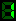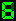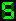# sRGB/XYZ/L*a*b*/CIELAB/ICCLAB/ITULABColor Space Conversion

Fill in one row, then select that as the starting point. The other rows will be computed:
 Startedfrom Computefrom sRGB88-bit sRGB [0,255] R = G = B = sRGB'Non-linear sRGB [0,1] R' = G' = B' = sRGB [0,1] R = G = B = CIEXYZ [0,1] X = Y = Z = L*a*b* L* = a* = b* = CIELAB88-bit CIELAB L8 = [0,255] a8 = [-127,127] b8 = [-127,127] ICCLAB88-bit ICCLAB L8 = [0,255] a8 = [0,255] b8 = [0,255] ITULAB88-bit ITULAB L8 = [0,255] a8 = [0,255] b8 = [0,255] ITULAB gamut Lmin = Lmax = amin = amax = bmin = bmax =

## Computation PathFor details on the conversion, please see Eugene Vishnevsky's conversion forumlas.